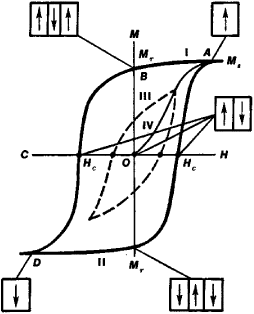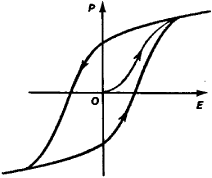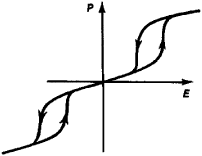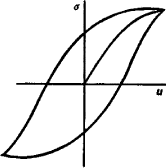# hysteresis

(redirected from hysteretic)
Also found in: Dictionary, Thesaurus, Medical, Financial, Wikipedia.
Related to hysteretic: Hysteresis loop, Hysteresis loss

## hysteresis

(hĭs'tərē`sĭs), phenomenon in which the response of a physical system to an external influence depends not only on the present magnitude of that influence but also on the previous history of the system. Expressed mathematically, the response to the external influence is a doubled-valued function; one value applies when the influence is increasing, the other applies when the influence is decreasing. Magnetic hysteresis occurs when a permeable material like soft iron is magnetized by being subjected to an external magnetic field. The induced magnetization tends to lag behind the magnetizing force. If a field is applied to an initially unmagnetized sample and is then removed, the sample retains a residual magnetization (it has become a permanent magnet). The graph of the magnetic induction B versus the magnetic field H is called a hysteresis loop. The area of the loop is proportional to the energy dissipated as heat when the system goes through a cycle; this represents a considerable energy loss in alternating-current machinery. Thermal hysteresis occurs when the value of a given property of a body depends not only on the body's temperature but also on whether the temperature is rising or falling. An example is the dielectric constant versus temperature for certain crystals. Another kind of hysteresis is a common feature of control or cybernetic systems. A familiar example is a thermostat controlling a source of heat and set at some temperature T0. When the room temperature falls through T0 to some lower temperature T1, the heating power is switched on. When the room temperature rises through T0 to some higher temperature T2, the power is switched off. Thus, for temperatures lower than T1, the heat is always on; for temperatures higher than T2, the heat is always off; but for temperatures between T1 and T2, the heat may be on or off (double-valued response), depending on which of the two temperatures T1 and T2 occurred most recently in the system's history. Unlike the previous examples, this hysteresis effect is not naturally occurring; it is designed into the control system to prevent the damage to the system that would arise from switching on and off too frequently.

## Hysteresis

A phenomenon wherein two (or more) physical quantities bear a relationship which depends on prior history. More specifically, the response Y takes on different values for an increasing input X than for a decreasing X.

If one cycles X over an appropriate range, the plot of Y versus X gives a closed curve which is referred to as the hysteresis loop. The response Y appears to be lagging the input X.

Hysteresis occurs in many fields of science. Perhaps the primary example is of magnetic materials where the input variable H (magnetic field) and response variable B (magnetic induction) are traditionally chosen. For such a choice of conjugate variables, the area of the hysteresis loop takes on a special significance, namely the conversion of energy per unit volume to heat per cycle. For mechanical hysteresis, it is customary to take the variables stress and strain, where the energy density loss per cycle is related to the internal friction. Thermal hysteresis is characteristic of many systems, particularly those involving phase changes, but here the hysteresis loops are not usually related to energy loss. See Ferroelectrics

McGraw-Hill Concise Encyclopedia of Physics. © 2002 by The McGraw-Hill Companies, Inc.
The following article is from The Great Soviet Encyclopedia (1979). It might be outdated or ideologically biased.

## Hysteresis

a phenomenon that consists in the fact that a physical quantity that characterizes the state of a body (for example, magnetization) depends nonuniquely on the physical quantity that characterizes external conditions (for example, a magnetic field). Hysteresis is observed in cases in which the state of a body at a given instant is determined by external conditions not only at that instant but also at preceding instants. The nonunique dependence of quantities is observed in any process, since a certain amount of time (the relaxation time) is always required for a change in the state of a body, and the reaction of the body lags behind the factors responsible for the change. The slower the change in external conditions, the smaller the lag. However, for some processes the lag does not diminish as the change in external conditions becomes slower. In these cases the nonunique dependence of the quantities is called a hysteretic relationship, and the phenomenon itself is called hysteresis.

Hysteresis is observed in various substances and physical processes. Of greatest interest are magnetic, dielectric, and elastic hysteresis.

Magnetic hysteresis Magnetic hysteresis is observed in magnetic materials, such as ferromagnets. The main feature of ferromagnets is the presence of spontaneous magnetization. A ferromagnet usually is not uniformly magnetized but is divided into domains—regions of uniform spontaneous magnetization whose degree of magnetization (the magnetic moment per unit volume) is identical, although the directions are different. Under the effect of an external magnetic field the number and size of the domains magnetized along the field increase at the expense of other domains. Moreover, the magnetic moments of certain domains may rotate in the direction of the field. As a result the magnetic moment of the sample increases.

The dependence of the magnetic moment M of a ferromagnetic sample on the intensity H of the external magnetic field (the magnetization curve) is shown in Figure 1. In a sufficiently strong magnetic field the sample is magnetized to saturation (as the field increases further, the value of M remains virtually unchanged—point A). Here the sample consists of one domain with a magnetic moment of saturation Ms oriented along the field. As the intensity H of the external magnetic field is reduced, the magnetic moment M of the sample will decline along curve I primarily because of the appearance and growth of domains whose magnetic moment is oriented against the field. The growth of the domains is due to the movement of the domain walls. This movement is hindered by the presence in the sample of various defects (such as impurities or inhomogeneities) that strengthen the domain walls at some points; very strong magnetic fields are required to displace them. Therefore as the field H drops to zero, the so-called residual magnetic moment Mr (point B) is retained. A sample can be completely demagnetized only in a sufficiently strong field of opposite direction, which is called a coercive field (coercive force) Hc (point C). As the magnetic field of reverse orientation is further increased, the sample is once again magnetized along the field to saturation (point D). Magnetic reversal (from point D to point A) takes place along curve II. Thus, as the field undergoes a cyclical change, the curve characterizing the change in the magnetic moment of the sample forms a magnetic hysteresis loop. If the field H changes cyclically with such limits that magnetization does not reach saturation, a nonlimiting magnetic hysteresis loop is produced (curve III). By reducing the extent of the change in field H to zero, the sample can be completely demagnetized (point O can be reached). The magnetization of the sample from point O proceeds along curve IV.Figure 1. Magnetic hysteresis loop for a ferromagnet: H is the intensity of the magnetic field, M is the magnetic moment of the sample, Hc is the coercive field, Mr is the residual magnetic moment, and Ms is the saturation magnetic moment. The nonlimiting hysteresis loop is shown by the dotted line. The domain structure of the sample for certain points on the loop is shown schematically.

In magnetic hysteresis different values of the magnetic moment M correspond to the same value of the external magnetic field intensity H. This nonuniqueness is due to the influence of the states of the sample that precede the given state (that is, to the magnetic prehistory of the sample).

The shape and size of magnetic hysteresis loops and the quantity Hc may range within wide limits in various ferromagnets. For example, in pure iron, Hc= 1 oersted, and in a magnico alloy Hc= 580 oersteds. A magnetic hysteresis loop is strongly affected by processing of the material, during which the number of defects is changed (Figure 2).

The area of a magnetic hysteresis loop is equal to the energy lost in the sample in one cycle of field change. This energy ultimately is used to heat the sample. Such energy losses are called hysteresis losses. In cases when losses to hysteresis are undesirable (for example, in transformer cores and in the stators and rotors of electrical machinery), magnetically soft materials with a low Hc and a small hysteresis loop area are used. On the other hand, magnetically hard materials with a high Hc are required to manufacture permanent magnets.

As the frequency of the alternating magnetic field (the number of magnetic reversal cycles per unit time) increases, other losses caused by eddy currents and magnetic viscosity are added to hysteresis losses. At high frequencies the area of the hysteresis loop increases correspondingly. Such a loop is sometimes called a dynamic loop, in contrast to the static loop described above.

Many other properties of a ferromagnet, such as electrical resistance and mechanical deformation, depend on the magnetic moment. A change in magnetic moment also brings about a change in these properties—for example, gal-vanomagnetic and magnetostrictive hysteresis, respectively, are observed.

Dielectric hysteresis. Dielectric hysteresis is usually observed in ferroelectrics such as barium titanate. The dependence of the polarization P on the electrical field intensity E in ferroelectrics (Figure 3) is similar to the dependence of M on H in ferromagnets and is due to the presence of spontaneous electrical polarization and electrical domains and to the difficulty of rearranging the domain structure. Hysteresis losses make up a large part of dielectric losses in ferroelectrics.

Insofar as other characteristics of ferroelectrics such as deformation are associated with polarization, other types of hysteresis, such as piezoelectric hysteresis (Figure 4) and the hysteresis of the electrooptical effect, are related to dielectric hysteresis. In some cases double dielectric hysteresis loops are observed (Figure 5). This is due to the fact that a phase transition involving rearrangement of the crystalline structure takes place in the sample under the influence of the electrical field. This type of dielectric hysteresis is closely related to the hysteresis during phase transitions.Figure 2. Effect of mechanical and thermal treatment on the form of the magnetic hysteresis loop for permalloy: (1) after cold hardening, (2) after annealing, (3) curve for soft iron (for comparison)Figure 3. Dielectric hysteresis loop in a ferroelectric: P is the polarization of the sample, and E is the intensity of the electrical fieldFigure 4. Hysteresis loop of the reverse piezoelectric effect in barium titanate: U is the deformation, and E is the intensity of the electrical fieldFigure 5. Double dielectric hysteresis loopFigure 6. Elastic hysteresis loop: σ is the mechanical stress, and u is the deformation

Elastic hysteresis. Elastic hysteresis, or the hysteretic dependence of the deformation u on the mechanical stress σ, is observed in any real materials for sufficiently great stress (Figure 6). Elastic hysteresis occurs whenever a plastic (inelastic) deformation occurs. A plastic deformation results from the displacement of defects, such the dislocations that are always present in real materials. Impurities, inclusions, and other defects, as well as the crystal lattice itself, tend to hold a dislocation in certain positions in the crystal. Therefore, stresses of sufficient magnitude to shift the dislocation are required. Machining and the introduction of impurities lead to the pinning of dislocations, as a result of which strengthening of the material occurs. Plastic deformation and elastic hysteresis are observed at greater stresses. The energy loss in the sample in one cycle ultimately goes to heat the object. Losses to elastic hysteresis contribute to internal friction. In the case of elastic deformations there are, in addition to hysteresis losses, other losses such as those caused by viscosity. The magnitude of these losses, in contrast to hysteresis losses, depends on the frequency of change of σ (or u).The concept of elastic hysteresis is sometimes used more broadly—for example, in terms of a dynamic elastic hysteresis loop, which includes all losses at a given frequency.

### REFERENCES

Kirenskii, L. V. Magnetizm, 2nd ed. Moscow, 1967.
Vonsovskii, S. V. Sovremennoe uchenie o magnetizme. Moscow-Leningrad, 1952.
Bozorth, R. Ferromagnetizm. Moscow, 1956. (Translated from English.)
Jona, F., and G. Shirane. Segnetoelektricheskie kristally. Moscow, 1965. (Translated from English.)
Postnikov, V. S. Vnutrennee trenie v metallakh. Moscow, 1969.
Fizicheskii entsiklopedicheskii slovar’, vol. 1. Moscow, 1960.

A. P. LEVANIUK and D. G. SANNIKOV

## hysteresis

[‚his·tə′rē·səs]
(electronics)
An oscillator effect wherein a given value of an operating parameter may result in multiple values of output power or frequency.
(electromagnetism)
(nucleonics)
A temporary change in the counting-rate-voltage characteristic of a radiation counter tube, caused by its previous operation.
(physics)
The dependence of the state of a system on its previous history, generally in the form of a lagging of a physical effect behind its cause.
McGraw-Hill Dictionary of Scientific & Technical Terms, 6E, Copyright © 2003 by The McGraw-Hill Companies, Inc.

## hysteresis

The lag between making a change, such as increasing or decreasing power, and the response or effect of that change. It typically refers to turn-on and turn-off points in electrical, electronic and mechanical systems. For example, if a thermostat set for 70 degrees turns on when the temperature reaches 68 and turns off at 72, the hysteresis is the range from 68 to 72.
Copyright © 1981-2019 by The Computer Language Company Inc. All Rights reserved. THIS DEFINITION IS FOR PERSONAL USE ONLY. All other reproduction is strictly prohibited without permission from the publisher.
References in periodicals archive ?
First and foremost, Figure 2 shows the comparison of hysteretic curves of specimen SW4.
Fan, "Experimental study and analytical modeling on hysteretic behavior of plain concrete in uniaxial cyclic tension," International Journal of Fatigue, vol.
An alternative hypothesis, based on the dithiol/disulfide redox motif of the Grx-like domain, was put forward to explain the hysteretic behavior of the enzyme .
where [F.sub.h] is an additional nonlinear hysteretic suspension damping and stiffness force, which is dependent on the relative displacement and velocity:
This restriction can be observed for hysteretic SHLC components measured in the last three (213.08 V, 218.26 V, 220.00 V) corresponding measurement points (Fig.
Block Diagram of the Thermal Shutdown Circuitry with hysteretic characteristic implemented by the current
By increasing [rho], the maximum value of the hysteretic output reaches to unity asymptotically.
Tan [delta] is the ratio of G" to G' and is a measure of the loss-rate of mechanical energy in a hysteretic system.
According to a nonlinear hysteretic piano hammer model and in reality , the duration of contact between the hammer and the string decreases with the increasing of the striking velocity of the hammer.
A long-standing interest has been with the modelling of automotive hydromounts--notoriously non-linear systems, where new techniques are being developed for piecewise linear and hysteretic systems, which work when traditional methods fail.
Test frequency was decreased with increasing stress level in order to avoid the excessive hysteretic heating that causes the specimens to fail due to permanent plastic deformation rather than fracture.
After reviewing nonlinear acoustics in homogeneous media, Nazarov and Radostin present results of their theoretical and experimental research on nonlinear processes caused by the propagation and interaction of longitudinal elastic waves in micro-inhomogeneous media with a strong acoustic nonlinearity of different types--elastic, hysteretic, and non-elastic.

Site: Follow: Share:
Open / Close